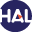Home page > 12. Volume 7 N°2 (2010) > Short Notes: Kinetic energy control in explicit Finite Volume (...)

# Short Notes: Kinetic energy control in explicit Finite Volume discretizations of the incompressible and compressible Navier-Stokes equations

Friday 19 November 2010,

Abstract: We prove that, under a cfl condition, the explicit upwind finite volume discretization of the convection operator C(u) = ∂t(ρu) + div(uq), with a given density ρ and momentum q, satisfies a discrete kinetic energy decrease property, provided that the convection operator satisfies a ”consistency-with-the-mass-balance property”, which can be simply stated by saying that it vanishes for a constant advected field u.

Key words : Compressible Navier-Stokes equations, Finite Volume discretizations, Stability, Kinetic Energy.

Paper presented by: Jean-Marc HérardVoir sur HAL : https://hal.archives-ouvertes.fr/hal-01112391